Question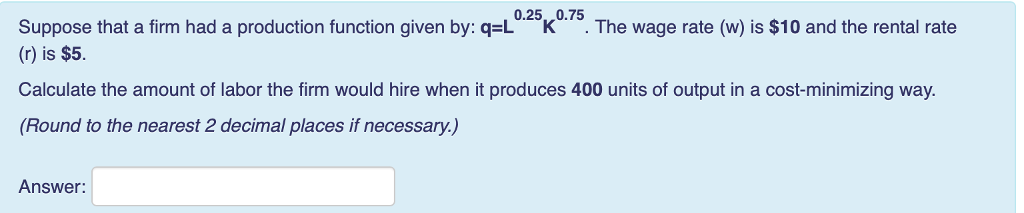A firm produces output in the cost minimizing way when it used that quantity of two inputs (Labor and capital) at which following condition is fulfilled -

MPL/MPK = PL/PK

Production function is as follows -

q = L0.25K0.75

Calculate MPL -

MPL = dq/dL

MPL = d(L0.25K0.75)/dL

MPL = 0.25K0.75/L0.25

MPK = dq/dK

MPK = d(L0.25K0.75)/dK

MPK = 0.75L0.25/K0.25

Cost minimizing condition is as follows -

MPL/MPK = PL/PK

(0.25K0.75/L0.25)/(0.75L0.25/K0.25) = 10/5

0.25K/0.75L = 2

K/3L = 2

K = 6L

Firm is producing 400 units of output.

So,

q = L0.25K0.75

400 = L0.25K0.75

400 = L0.25(6L)0.75

400 = 60.75 L0.25 L0.75

400 = 3.83 * L

L = 400/3.83 = 104.44

Thus,

The firm will use 104.44 units of labor.

#### Earn Coins

Coins can be redeemed for fabulous gifts.

Similar Homework Help Questions
• ### Suppose that a firm had a production function given by q-21 K The rental rate for...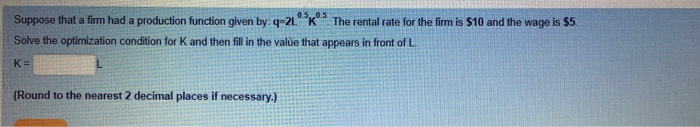Suppose that a firm had a production function given by q-21 K The rental rate for the fim is \$10 and the wage is s5 Solve the optimization condition for K and then fill in the value that appears in front of L K- Round to the nearest 2 decimal places if necessary.) Suppose that a firm had a production function given by q-L025k075. The wage rate (w) is \$10 and the rental rate () is \$10 Calculate the amount...

• ### Suppose that a firm had a production function given by: q=L^0.25*K^0.75. The wage rate (w) is...

Suppose that a firm had a production function given by: q=L^0.25*K^0.75. The wage rate (w) is \$10 and the rental rate (r) is \$20. Calculate the amount of labor the firm would hire when it produces 300 units of output in a cost-minimizing way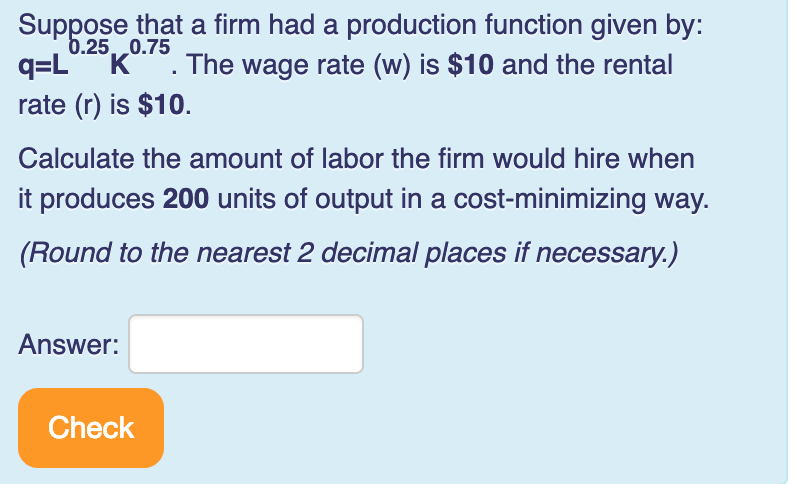Please help me! Thank you! Suppose that a firm had a production function given by: q-L K. The wage rate (w) is \$10 and the rental rate (r) is \$10 Calculate the amount of labor the firm would hire when t produces 200 units of output in a cost-minimizing way. .25, 0.75 (Round to the nearest 2 decimal places if necessary.) Answer: Check

• ### Question 10 Tries remaining: 2 Suppose that a firm had a production function given by: q=Lºkº....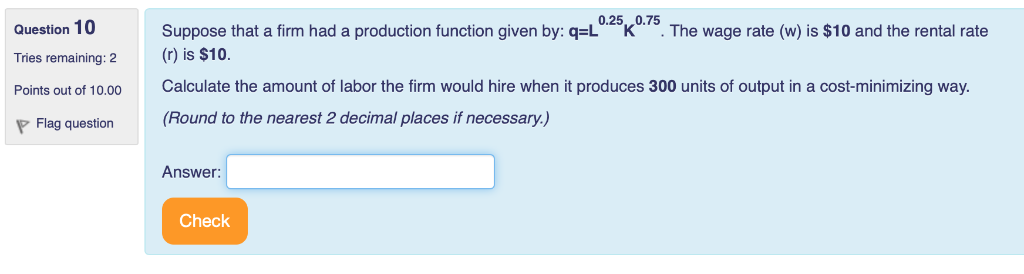Question 10 Tries remaining: 2 Suppose that a firm had a production function given by: q=Lºkº. The wage rate (w) is \$10 and the rental rate () is \$10. Calculate the amount of labor the firm would hire when it produces 300 units of output in a cost-minimizing way. (Round to the nearest 2 decimal places if necessary.) Points out of 10.00 P Flag question Answer: Check

• ### Suppose that a firm has a production function given by: ? = ?^?.???^?.? . The wage...

Suppose that a firm has a production function given by: ? = ?^?.???^?.? . The wage rate is \$18 and the rental rate is \$9. 12. Suppose that the firm has 4 units of capital in the short run. Find the short run total cost function. ________________________________ 13. Find the marginal product of labor (MPL) function. ________________________________ 14. Solve the optimization condition for K and write that equation. ________________________________ 15. Suppose the firm wants to minimize the cost of producing...

• ### Question 5 Tries remaining: 2 Points out of 10.00 Suppose you own an acre of land....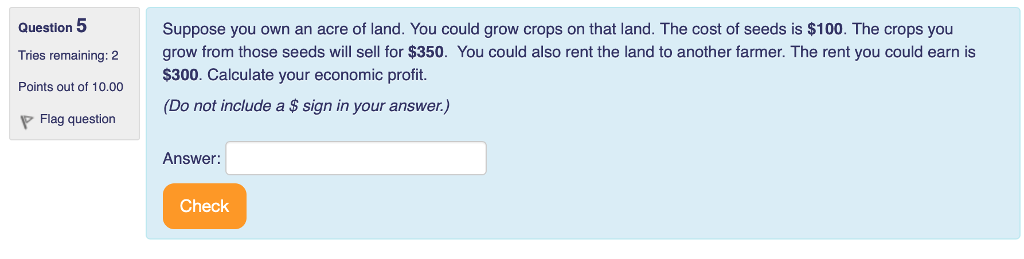Question 5 Tries remaining: 2 Points out of 10.00 Suppose you own an acre of land. You could grow crops on that land. The cost of seeds is \$100. The crops you grow from those seeds will sell for \$350. You could also rent the land to another farmer. The rent you could earn is \$300. Calculate your economic profit. (Do not include a \$ sign in your answer.) Flag question Answer: Check Question 6 Tries remaining: 2 Points out...

• ### Question 9 0.5,,0.25 Suppose that a firm had a production function given by: q-2L K ....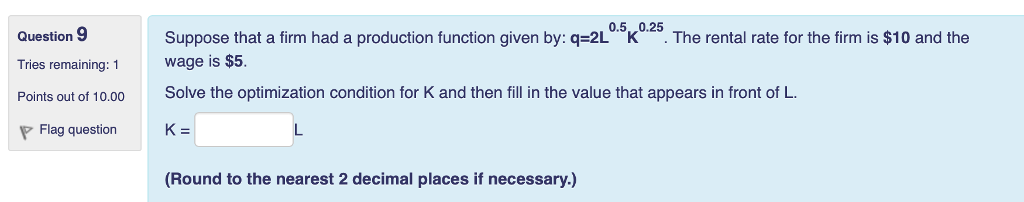Question 9 0.5,,0.25 Suppose that a firm had a production function given by: q-2L K . The rental rate for the firm is \$10 and the Wage is \$5. Solve the optimization condition for K and then fill in the value that appears in front of L. Tries remaining: 1 Flag question K (Round to the nearest 2 decimal places if necessary.)

• ### Suppose the production function for a firm is given by: q-2L *4K. If the firm currently...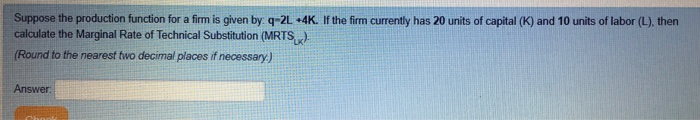Suppose the production function for a firm is given by: q-2L *4K. If the firm currently has 20 units of capital (K) and 10 units of labor (L), then calculate the Marginal Rate of Technical Substitution (MRTSx) (Round to the nearest fwo decimal places if necessary) Answer Suppose the production function for a firm is given by: q-4L K 25 If the firm currently has 20 units of capital (K) and 10 units of labor (L), then calculate the Marginal...

• ### Suppose the production function for a firm is given by: q 8L +2K. If the firm...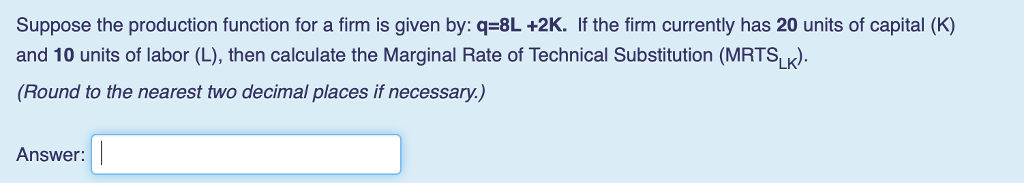Suppose the production function for a firm is given by: q 8L +2K. If the firm currently has 20 units of capital (K) and 10 units of labor (L), then calculate the Marginal Rate of Technical Substitution (MRTSL). (Round to the nearest two decimal places if necessary.) Answer:

• ### Suppose the production function for a firm is given by: q=4L0.5K0.25. If the firm currently has...

Suppose the production function for a firm is given by: q=4L0.5K0.25. If the firm currently has 10 units of capital (K) and 10 units of labor (L), then calculate the Marginal Rate of Technical Substitution (MRTSLK). (Round to the nearest two decimal places if necessary.)